# Thank You, Northside Donors

Many thanks to you, our generous and supportive community, as we met our match challenge and raised more than \$60,000 for Northside!

However we have not reached our overall goal for the Annual Appeal. Can you help? It's not too late. Every dollar counts.

Monetary contributions, employer matching gifts, and gifts of stock are always welcome in our Annual Appeal to raise funds for Northside. Contact Friends of Northside HERE to find out how to turn your shares of stock into a gift to Northside.

 table.module-3{width:38.49%;padding:0}table div table+table+table+table div table{width:38.49%;float:none;margin-left:auto;margin-right:auto;padding:0}table div table+table+table+table div table a{border:0 none;text-decoration:none}table div table+table+table+table div table img{width:100%!important;border:0 none;text-decoration:none}table div table+table+table+table div table td{width:100%;padding:0}/* styles */
 table div table+table+table+table+table div table{width:100%;padding:0}table div table+table+table+table+table div table img{width:96.23%;padding:0;float:none}table div table+table+table+table+table div table td{width:100%;padding:0 1.88% 18px}/* styles */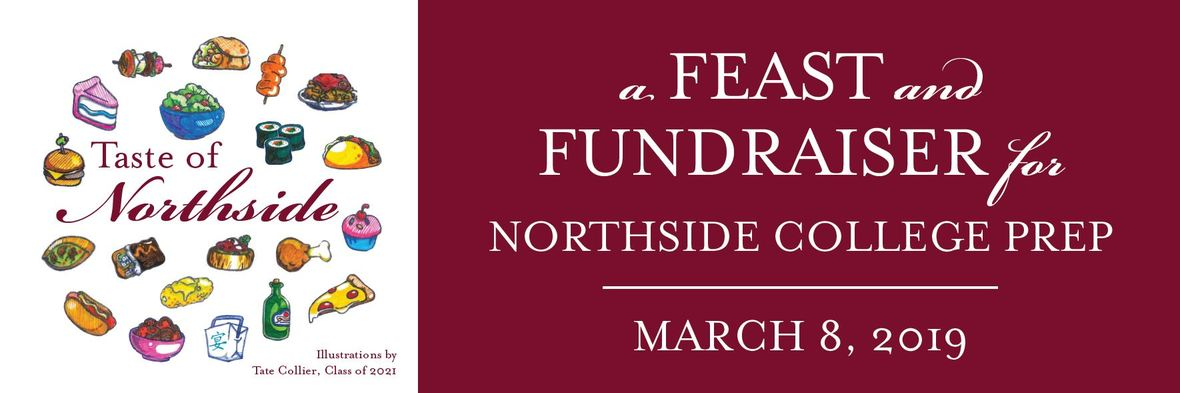# TASTE OF NORTHSIDE - a FEAST and FUNDRAISER for Northside College Prep

## Spring Benefit & Online Auction

There are so many ways to contribute to Northside, and we encourage you to save Friday, March 8 for Northside's annual spring benefit, Taste of Northside, at the DANK Haus. Invitations will be in the mail soon. It’s a wonderful night of celebration, community, and fun. Please join us!# Call for Auction Donations

It’s auction time! This major fundraiser raises critical funds to support Northside. Please help us gather items to include in our online silent auction. Some ideas include:

Sports Tickets: Bears, Bulls, Hawks, Cubs, Sox, Fire, College Teams, or more

Restaurant Gift Cards: Who doesn't love to eat out?

Hotels and Getaways: Local hotels, an apartment in Paris, your home in Michigan, Indiana, or Wisconsin... Also hotels and/or homes in college destinations would be great – Boston, New York, LA, etc.

Unique Experiences: Access to the inaccessible! Backstage pass, on-ice at the Blackhawks, sold-out shows, chef's dinner, Behind the Scenes...

Important Auction Dates:
Online Auction: March 1 - 10
Live Auction at the Party: March 8

Kristen Werries Collier, kristenwerriescollier@gmail.com.

Click HERE for the donation form. Print a form and carry it with you. Ask your favorite stores, theaters, and more if they will contribute an item to our auction. It takes just a few minutes, and all dollars raised in the auction make a difference for our school.

You can drop off auction items at school or email the committee HERE. Deadline for submissions is February 16.Corporate Sponsorship contributions will be used to underwrite the cost of the 2019 Spring Benefit and enable Friends of Northside to provide more of its financial resources for programs and services for the school not covered by the CPS budget. For sponsorship opportunities and in-kind donations, please contact Matt Schechter, matthew.d.schechter@gmail.com. Click HERE for the sponsorship form.We are putting together a slideshow of our students participating in the many activities that make Northside special. If you have pictures you'd like to be considered for inclusion in the presentation, please forward them to Debra Swan at debra@theswans.net.

## Food & Beverages Needed

Have a connection to a local restaurant or beverage vendor? We need items to add to our dinner buffet, as well as wine, champagne, and soda. Contact Debra Swan, debra@theswans.net, if you can help.

## Build a Social Experience for NCP

A fun aspect of our annual Benefit fundraising effort each year is the Parent Parties! Northside families offer to host a party for other Northside parents and families. The host pays for the party, guests pay to attend and the revenue generated from tickets is all donated to Northside. These parties raise \$500-\$2500 each and are a great opportunity to meet and mingle with other Northside parents. A few ideas are Moms & Margaritas, Poker Night, Wine Tasting, Backyard BBQ, Theme Dinner Party, and more.

Please consider hosting a party like one of those suggested, come up with your own idea or we can help you put something together. Reach out to Nancy Liljedahl at n.liljedahl@comcast.net if you are interested in hosting or want to brainstorm. Thanks for your support!

 table div table+table+table+table+table+table+table+table+table+table+table+table+table+table div table{width:100%;padding:0}table div table+table+table+table+table+table+table+table+table+table+table+table+table+table div table img{width:96.23%;padding:0;float:none}table div table+table+table+table+table+table+table+table+table+table+table+table+table+table div table td{width:100%;padding:0 1.88% 18px}/* styles */# Homecoming & Spirit Week

January 14 - 18: A week of school festivities!
The NCP Student Council has planned a fun week for our students!

## Spirit Week Wear

Best outfit each day will win a free ticket to Homecoming!

▪ Meme Monday
▪ Twin Tuesday
▪ Class War Wednesday – wear class color (9th- white, 10th- gray, 11th- black, 12th-maroon)
▪ Pajama Thursday
▪ Vacation Friday
 ▪ Meme Monday
 ▪ Twin Tuesday
 ▪ Class War Wednesday – wear class color (9th- white, 10th- gray, 11th- black, 12th-maroon)
 ▪ Pajama Thursday
 ▪ Vacation Friday

Speaking of “Spirit Wear” the NCP Booster Club is hosting a Spirit Wear resale.Tickets go on sale Monday, January 14

▪ NCP Student - \$10
▪ Non-NCP Guests - \$15
 ▪ NCP Student - \$10
 ▪ Non-NCP Guests - \$15

For Non-NCP Guests, parents must sign a permission form.

Tuesday, January 15 – Women's Games NCP vs Michelle Clark
5:00 pm (V); 6:30 pm (JV)

Wednesday, January 16 – Men's Games NCP vs Payton
Concession Stand Opens at 4:00 pm
Alina Yamin Recognition Ceremony at Half Time
JV game begins at 6:30 pm

## 2000 Points Recognition Ceremony

During half-time on Wednesday, January 16
Signed Game Ball presented to Alina Yamin class of 2019
She was recently named to the all-tournament team. We are so proud of her!
Come on out to support this amazing accomplishment!

NCP Poms Team to Perform at Half Time!

## Retro Team Uniform Resale

Wednesday, January 16

Stop by and pick up second-hand athletic wear for a steal! Items priced from \$1-\$5. Proceeds go to the NCP Booster Club.

 table div table+table+table+table+table+table+table+table+table+table+table+table+table+table+table+table+table+table+table+table div table{width:100%;padding:0}table div table+table+table+table+table+table+table+table+table+table+table+table+table+table+table+table+table+table+table+table div table img{width:96.23%;padding:0;float:none}table div table+table+table+table+table+table+table+table+table+table+table+table+table+table+table+table+table+table+table+table div table td{width:100%;padding:0 1.88% 18px}/* styles */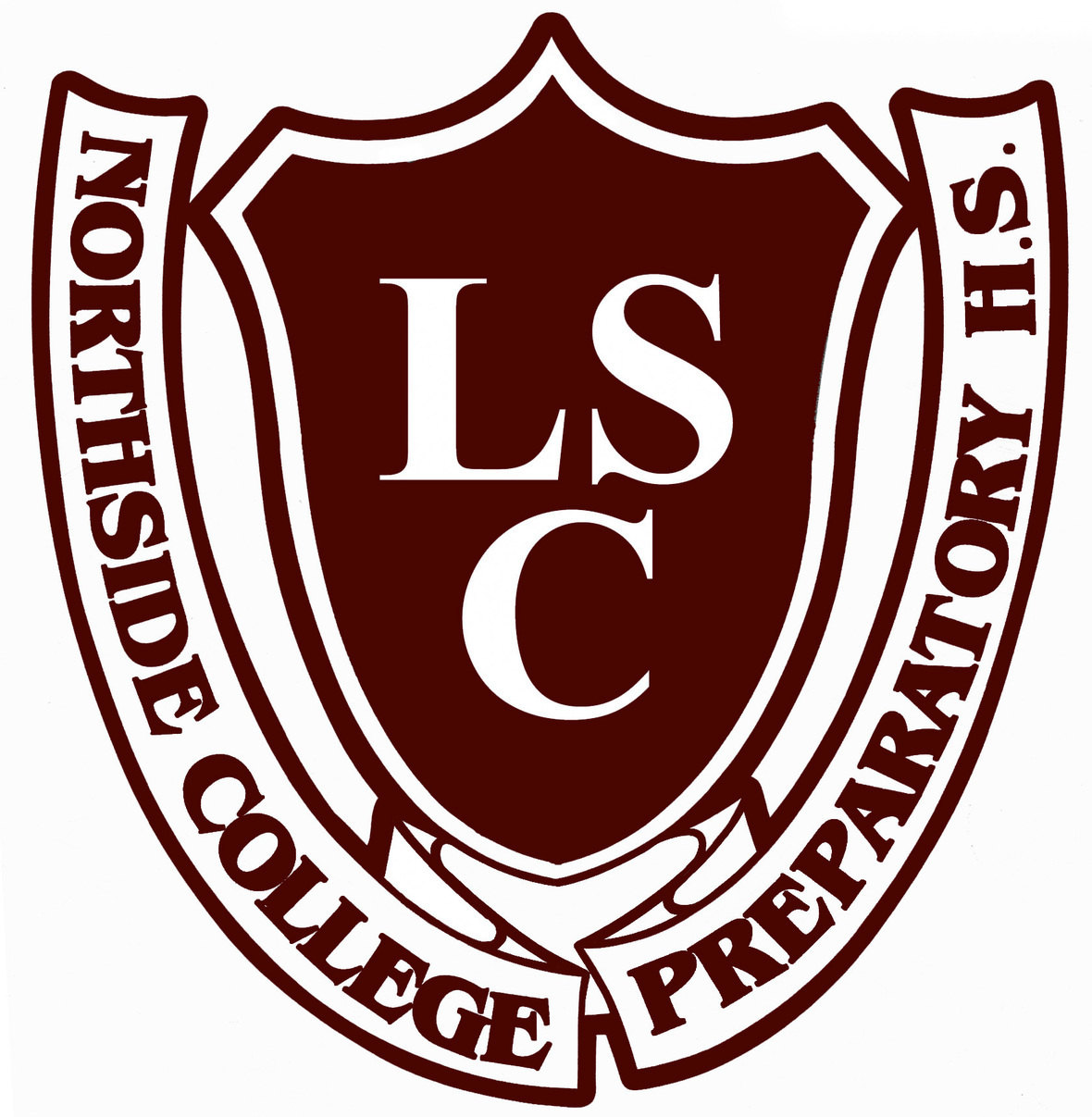# LSC Meeting

Tuesday, January 15
7:00 pm
Faculty Lounge

 table div table+table+table+table+table+table+table+table+table+table+table+table+table+table+table+table+table+table+table+table+table+table div table{width:100%;padding:0}table div table+table+table+table+table+table+table+table+table+table+table+table+table+table+table+table+table+table+table+table+table+table div table img{width:96.23%;padding:0;float:none}table div table+table+table+table+table+table+table+table+table+table+table+table+table+table+table+table+table+table+table+table+table+table div table td{width:100%;padding:0 1.88% 18px}/* styles */# Beginning Band Concert

Tuesday, January 15
7:00 - 10:00 pm

 table div table+table+table+table+table+table+table+table+table+table+table+table+table+table+table+table+table+table+table+table+table+table+table+table div table{width:100%;padding:0}table div table+table+table+table+table+table+table+table+table+table+table+table+table+table+table+table+table+table+table+table+table+table+table+table div table img{width:96.23%;padding:0;float:none}table div table+table+table+table+table+table+table+table+table+table+table+table+table+table+table+table+table+table+table+table+table+table+table+table div table td{width:100%;padding:0 1.88% 18px}/* styles */# This Week in Sports

Come to a game and support our student athletes!

▪ Tuesday, January 15 at 5:00 pm (V) and 6:30 pm (SO) vs. Michelle Clark at NCP
 ▪ Tuesday, January 15 at 5:00 pm (V) and 6:30 pm (SO) vs. Michelle Clark at NCP

▪ Wednesday, January 16 at 5:00 pm (V) and 6:30 pm (SO) vs. Payton College Prep at NCP
▪ Friday, January 18 at 5:00 pm (V) and 6:30 pm (SO) vs. Von Steuben at Von Steuben
 ▪ Wednesday, January 16 at 5:00 pm (V) and 6:30 pm (SO) vs. Payton College Prep at NCP
 ▪ Friday, January 18 at 5:00 pm (V) and 6:30 pm (SO) vs. Von Steuben at Von Steuben

## Women’s Bowling

▪ Monday, January 14 at 4:15 pm vs. Taft HS at Home (Waveland Bowl)
 ▪ Monday, January 14 at 4:15 pm vs. Taft HS at Home (Waveland Bowl)

## Men’s & Women’s Indoor Track & Field

Indoor season will begin on Monday, January 14. Contact Director of Athletics Henderson for details.

 table div table+table+table+table+table+table+table+table+table+table+table+table+table+table+table+table+table+table+table+table+table+table+table+table+table+table+table div table{width:100%;padding:0}table div table+table+table+table+table+table+table+table+table+table+table+table+table+table+table+table+table+table+table+table+table+table+table+table+table+table+table div table img{width:96.23%;padding:0;float:none}table div table+table+table+table+table+table+table+table+table+table+table+table+table+table+table+table+table+table+table+table+table+table+table+table+table+table+table div table td{width:100%;padding:0 1.88% 18px}/* styles */# No School

Monday, January 21
In observance of Martin Luther King Jr. Day

 table div table+table+table+table+table+table+table+table+table+table+table+table+table+table+table+table+table+table+table+table+table+table+table+table+table+table+table+table+table div table{width:100%;padding:0}table div table+table+table+table+table+table+table+table+table+table+table+table+table+table+table+table+table+table+table+table+table+table+table+table+table+table+table+table+table div table img{width:96.23%;padding:0;float:none}table div table+table+table+table+table+table+table+table+table+table+table+table+table+table+table+table+table+table+table+table+table+table+table+table+table+table+table+table+table div table td{width:100%;padding:0 1.88% 18px}/* styles */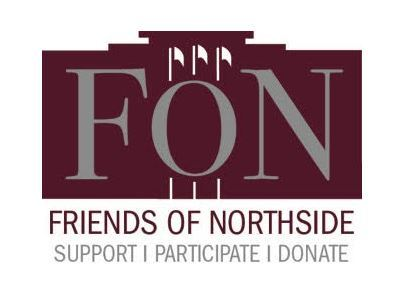# Friends of Northside Meeting

Tuesday, January 22
7:00 pm
Faculty Lounge

 table div table+table+table+table+table+table+table+table+table+table+table+table+table+table+table+table+table+table+table+table+table+table+table+table+table+table+table+table+table+table+table div table{width:100%;padding:0}table div table+table+table+table+table+table+table+table+table+table+table+table+table+table+table+table+table+table+table+table+table+table+table+table+table+table+table+table+table+table+table div table img{width:96.23%;padding:0;float:none}table div table+table+table+table+table+table+table+table+table+table+table+table+table+table+table+table+table+table+table+table+table+table+table+table+table+table+table+table+table+table+table div table td{width:100%;padding:0 1.88% 18px}/* styles */# Dates to Note

Monday, January 28 through Wednesday, January 30
Semester 1 Final Exams

Thursday, January 31
End of Quarter 2/Semester 1

Friday, February 1
School Improvement Day, no school for students

 table div table+table+table+table+table+table+table+table+table+table+table+table+table+table+table+table+table+table+table+table+table+table+table+table+table+table+table+table+table+table+table+table+table div table{width:100%;padding:0}table div table+table+table+table+table+table+table+table+table+table+table+table+table+table+table+table+table+table+table+table+table+table+table+table+table+table+table+table+table+table+table+table+table div table img{width:96.23%;padding:0;float:none}table div table+table+table+table+table+table+table+table+table+table+table+table+table+table+table+table+table+table+table+table+table+table+table+table+table+table+table+table+table+table+table+table+table div table td{width:100%;padding:0 1.88% 18px}/* styles */## Purchase Yearbook Personal Ads for Seniors

All materials are due by Friday, March 17.

Commemorate your student's accomplishments or declare your best wishes with a personal ad in the upcoming Stampede. The prices are:

▪ Full Page \$150
▪ Half Page \$80
▪ Quarter Page \$50

Members of the Stampede can create the ad for you, or you can submit a pre-made ad yourself (photo resolution no less than 600 dpi, please). If you choose to submit a pre-made ad, please also email the digital photos as separate attachments.

Questions? Contact Marion McCreedy, Stampede adviser, at mkmccreedy@cps.edu.## And While We're Talking About Yearbooks...

Order yours now to avoid the March 1 price increase!

Yearbooks were not included as an item on the school fee schedule this year.

2) Enter code 4187219

 table div table+table+table+table+table+table+table+table+table+table+table+table+table+table+table+table+table+table+table+table+table+table+table+table+table+table+table+table+table+table+table+table+table+table+table+table+table div table{width:100%;padding:0}table div table+table+table+table+table+table+table+table+table+table+table+table+table+table+table+table+table+table+table+table+table+table+table+table+table+table+table+table+table+table+table+table+table+table+table+table+table div table img{width:96.23%;padding:0;float:none}table div table+table+table+table+table+table+table+table+table+table+table+table+table+table+table+table+table+table+table+table+table+table+table+table+table+table+table+table+table+table+table+table+table+table+table+table+table div table td{width:100%;padding:0 1.88% 18px}/* styles */# Juniors - Class of 2020! Save the Dates!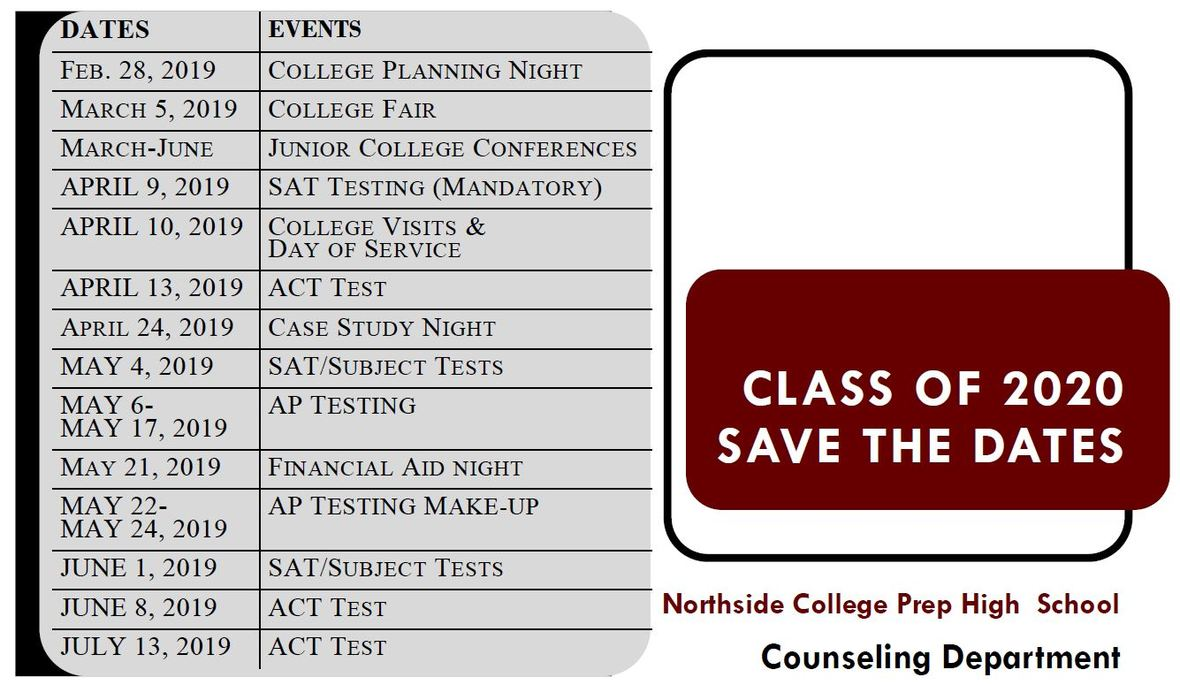table div table+table+table+table+table+table+table+table+table+table+table+table+table+table+table+table+table+table+table+table+table+table+table+table+table+table+table+table+table+table+table+table+table+table+table+table+table+table+table+table div table{width:100%;padding:0}table div table+table+table+table+table+table+table+table+table+table+table+table+table+table+table+table+table+table+table+table+table+table+table+table+table+table+table+table+table+table+table+table+table+table+table+table+table+table+table+table div table img{width:96.23%;padding:0;float:none}table div table+table+table+table+table+table+table+table+table+table+table+table+table+table+table+table+table+table+table+table+table+table+table+table+table+table+table+table+table+table+table+table+table+table+table+table+table+table+table+table div table td{width:100%;padding:0 1.88% 18px}/* styles */Ms. Woodruff is hoping to run a sewing colloquium this spring but needs sewing machines. If you have a sewing machine you can donate, please contact Ms. Woodruff at jwoodruff@cps.edu.

 table div table+table+table+table+table+table+table+table+table+table+table+table+table+table+table+table+table+table+table+table+table+table+table+table+table+table+table+table+table+table+table+table+table+table+table+table+table+table+table+table+table+table div table{width:100%;padding:0}table div table+table+table+table+table+table+table+table+table+table+table+table+table+table+table+table+table+table+table+table+table+table+table+table+table+table+table+table+table+table+table+table+table+table+table+table+table+table+table+table+table+table div table img{width:96.23%;padding:0;float:none}table div table+table+table+table+table+table+table+table+table+table+table+table+table+table+table+table+table+table+table+table+table+table+table+table+table+table+table+table+table+table+table+table+table+table+table+table+table+table+table+table+table+table div table td{width:100%;padding:0 1.88% 18px}/* styles */# Travel this Spring with Northside

## Spring Break 2019 Trip to Spain

Four amazing cities and more than 15 sites to visit, including Madrid, Granada, Sevilla, and Costa del Sol!

What is included? 8 days of beautiful and historical España! Airfare, all hotel stays, transportation-comfortable coach bus, tour guides, breakfast and dinner, etc.

Open to ALL students.

## Spring Break 2019 Trip to England

On this trip you will experience the distinct character that is London. Admire St Paul's Cathedral, Big Ben,The House of Parliament, and Buckingham Palace. We will also travel to Somerset and visit Stonehenge the United Kingdom's most mysterious landmark, Bath, Stratford (see where William Shakespeare was born), and Oxford.

See Ms. Fernandez or Ms. Vosnos or click HERE for an informational brochure.

## Spring Break 2019 Trip to the Grand Canyon

Students will have a chance to connect with the majesty of our National Park system. We will spend 5 nights in the Grand Canyon camping, hiking, stargazing, and learning wilderness skills. Students will participate in a second-semester colloquium to prepare them for the trip. Trip sponsors and chaperones include Ms. Qazi, Ms. Schaldenbrand, and Mr. Barry Smith. Approximate cost: \$1,000 (includes flight, food, and lodging).

 table div table+table+table+table+table+table+table+table+table+table+table+table+table+table+table+table+table+table+table+table+table+table+table+table+table+table+table+table+table+table+table+table+table+table+table+table+table+table+table+table+table+table+table+table div table{width:100%;padding:0}table div table+table+table+table+table+table+table+table+table+table+table+table+table+table+table+table+table+table+table+table+table+table+table+table+table+table+table+table+table+table+table+table+table+table+table+table+table+table+table+table+table+table+table+table div table img{width:96.23%;padding:0;float:none}table div table+table+table+table+table+table+table+table+table+table+table+table+table+table+table+table+table+table+table+table+table+table+table+table+table+table+table+table+table+table+table+table+table+table+table+table+table+table+table+table+table+table+table+table div table td{width:100%;padding:0 1.88% 18px}/* styles */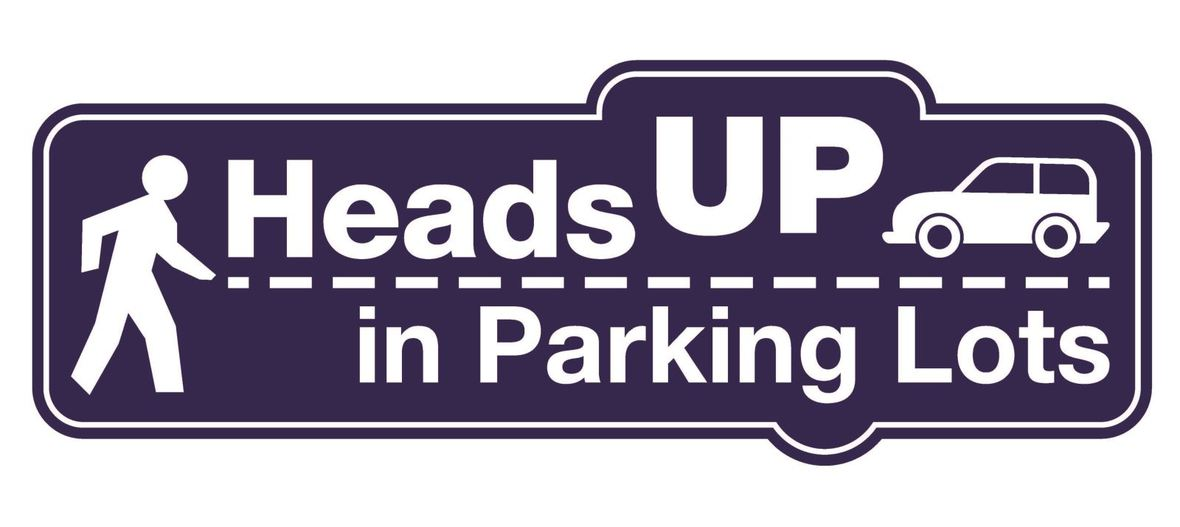# Parking Lot Safety and Procedures

Our NCP school parking lot is a busy place before and after school. Families dropping off and picking up students are asked to be mindful of both safety and courtesy. Please assist us by ensuring that traffic lanes stay clear during drop-off and pick-up times. Pulling over to the curb on the right as your student enters or exits your vehicle keeps students safe and traffic flow efficient.

Please remember that ALL VEHICLES MUST EXIT ONTO KEDZIE. You may enter the parking lot from Kedzie or Bryn Mawr, but regardless of where you enter, all cars must exit onto Kedzie. For the safety of our students and the rest of the Northside community, please do not exit onto Bryn Mawr.

 table div table+table+table+table+table+table+table+table+table+table+table+table+table+table+table+table+table+table+table+table+table+table+table+table+table+table+table+table+table+table+table+table+table+table+table+table+table+table+table+table+table+table+table+table+table+table+table div table{width:100%;padding:0}table div table+table+table+table+table+table+table+table+table+table+table+table+table+table+table+table+table+table+table+table+table+table+table+table+table+table+table+table+table+table+table+table+table+table+table+table+table+table+table+table+table+table+table+table+table+table+table div table img{width:96.23%;padding:0;float:none}table div table+table+table+table+table+table+table+table+table+table+table+table+table+table+table+table+table+table+table+table+table+table+table+table+table+table+table+table+table+table+table+table+table+table+table+table+table+table+table+table+table+table+table+table+table+table+table div table td{width:100%;padding:0 1.88% 18px}/* styles */# CPS Volunteer Requirements

According to CPS policy, anybody who is going to be in contact with students must be approved as a CPS volunteer. There are two levels of volunteers, Level 1 and Level 2.

## Level II Volunteers

(This process can be completed in a day & is required even of volunteers for Friends of Northside events)
A fingerprint criminal background check is not required for Level II Volunteers.

A Level II Volunteer is:

1. A parent providing supervised volunteer service in their child’s school or classroom for less than 10 hours/week;
2. Any individual providing volunteer service for less than 5 hours/week at a school where s/he does not have a child enrolled;
3. An individual providing incidental volunteer service with no ongoing individualized interaction with a student(s) including those who speak at a class/assembly, judge academic competitions, give musical performance, participate in the “Principal for a Day” program, job shadowing event or other one-time event provided where there is direct supervision of the activity/event by regular school employees;
4. A parent accompanying his/her child’s class on a one-day field trip or another type of extracurricular activity that does not involve an overnight stay; and/or
5. An individual providing volunteer service on projects/activities involving no or nominal contact with children (home-based volunteer activities, volunteers serving at Central or Network office).
 1 A parent providing supervised volunteer service in their child’s school or classroom for less than 10 hours/week;
 2 Any individual providing volunteer service for less than 5 hours/week at a school where s/he does not have a child enrolled;
 3 An individual providing incidental volunteer service with no ongoing individualized interaction with a student(s) including those who speak at a class/assembly, judge academic competitions, give musical performance, participate in the “Principal for a Day” program, job shadowing event or other one-time event provided where there is direct supervision of the activity/event by regular school employees;
 4 A parent accompanying his/her child’s class on a one-day field trip or another type of extracurricular activity that does not involve an overnight stay; and/or
 5 An individual providing volunteer service on projects/activities involving no or nominal contact with children (home-based volunteer activities, volunteers serving at Central or Network office).

## Level I Volunteers

(Please allow 8-12 weeks for the entire process)
A fingerprint criminal background check is required for all Level I Volunteers.

A Level I volunteer is:

1. A parent providing volunteer service in their child’s school or classroom for 10 or more hours/week;
2. An individual providing volunteer service for 5 or more hours/week at a school where s/he does not have a child enrolled; and/or
3. Any individual chaperoning an overnight school-sponsored trip, regardless of the hours/week that the volunteer serves (See also the Student Travel Policy).
 1 A parent providing volunteer service in their child’s school or classroom for 10 or more hours/week;
 2 An individual providing volunteer service for 5 or more hours/week at a school where s/he does not have a child enrolled; and/or
 3 Any individual chaperoning an overnight school-sponsored trip, regardless of the hours/week that the volunteer serves (See also the Student Travel Policy).

To become a volunteer, please visit CPS' Volunteer website and follow the instructions. There is also a link on Northside's website on the Parents page Northside Volunteers.

Assistant Principal Kaitlin O'Hare is Northside's Volunteer Coordinator. After applying to become a volunteer, you must visit the school and show Ms. O'Hare your ID, so she can approve you to move forward in the volunteer process. For Level II volunteers, that is the only step you must take. For Level I, you will receive further instructions from CPS.

 table div table+table+table+table+table+table+table+table+table+table+table+table+table+table+table+table+table+table+table+table+table+table+table+table+table+table+table+table+table+table+table+table+table+table+table+table+table+table+table+table+table+table+table+table+table+table+table+table+table div table{width:100%;padding:0}table div table+table+table+table+table+table+table+table+table+table+table+table+table+table+table+table+table+table+table+table+table+table+table+table+table+table+table+table+table+table+table+table+table+table+table+table+table+table+table+table+table+table+table+table+table+table+table+table+table div table img{width:96.23%;padding:0;float:none}table div table+table+table+table+table+table+table+table+table+table+table+table+table+table+table+table+table+table+table+table+table+table+table+table+table+table+table+table+table+table+table+table+table+table+table+table+table+table+table+table+table+table+table+table+table+table+table+table+table div table td{width:100%;padding:0 1.88% 18px}/* styles */table.module-49{width:94.34%;padding:0}table div table+table+table+table+table+table+table+table+table+table+table+table+table+table+table+table+table+table+table+table+table+table+table+table+table+table+table+table+table+table+table+table+table+table+table+table+table+table+table+table+table+table+table+table+table+table+table+table+table+table div table{width:94.34%;float:none;margin-left:auto;margin-right:auto;padding:0}table div table+table+table+table+table+table+table+table+table+table+table+table+table+table+table+table+table+table+table+table+table+table+table+table+table+table+table+table+table+table+table+table+table+table+table+table+table+table+table+table+table+table+table+table+table+table+table+table+table+table div table a{border:0 none;text-decoration:none}table div table+table+table+table+table+table+table+table+table+table+table+table+table+table+table+table+table+table+table+table+table+table+table+table+table+table+table+table+table+table+table+table+table+table+table+table+table+table+table+table+table+table+table+table+table+table+table+table+table+table div table img{width:100%!important;border:0 none;text-decoration:none}table div table+table+table+table+table+table+table+table+table+table+table+table+table+table+table+table+table+table+table+table+table+table+table+table+table+table+table+table+table+table+table+table+table+table+table+table+table+table+table+table+table+table+table+table+table+table+table+table+table+table div table td{width:100%;padding:0}/* styles */
 /* styles */ Check out The HoofBeat, Northside's online newspaper, to get NCP news from the student perspective!
 table div table+table+table+table+table+table+table+table+table+table+table+table+table+table+table+table+table+table+table+table+table+table+table+table+table+table+table+table+table+table+table+table+table+table+table+table+table+table+table+table+table+table+table+table+table+table+table+table+table+table+table+table div table{width:100%;padding:0}table div table+table+table+table+table+table+table+table+table+table+table+table+table+table+table+table+table+table+table+table+table+table+table+table+table+table+table+table+table+table+table+table+table+table+table+table+table+table+table+table+table+table+table+table+table+table+table+table+table+table+table+table div table img{width:96.23%;padding:0;float:none}table div table+table+table+table+table+table+table+table+table+table+table+table+table+table+table+table+table+table+table+table+table+table+table+table+table+table+table+table+table+table+table+table+table+table+table+table+table+table+table+table+table+table+table+table+table+table+table+table+table+table+table+table div table td{width:100%;padding:0 1.88% 18px}/* styles */table.module-52{width:57.17%;padding:0}table div table+table+table+table+table+table+table+table+table+table+table+table+table+table+table+table+table+table+table+table+table+table+table+table+table+table+table+table+table+table+table+table+table+table+table+table+table+table+table+table+table+table+table+table+table+table+table+table+table+table+table+table+table div table{width:57.17%;float:none;margin-left:auto;margin-right:auto;padding:0}table div table+table+table+table+table+table+table+table+table+table+table+table+table+table+table+table+table+table+table+table+table+table+table+table+table+table+table+table+table+table+table+table+table+table+table+table+table+table+table+table+table+table+table+table+table+table+table+table+table+table+table+table+table div table a{border:0 none;text-decoration:none}table div table+table+table+table+table+table+table+table+table+table+table+table+table+table+table+table+table+table+table+table+table+table+table+table+table+table+table+table+table+table+table+table+table+table+table+table+table+table+table+table+table+table+table+table+table+table+table+table+table+table+table+table+table div table img{width:100%!important;border:0 none;text-decoration:none}table div table+table+table+table+table+table+table+table+table+table+table+table+table+table+table+table+table+table+table+table+table+table+table+table+table+table+table+table+table+table+table+table+table+table+table+table+table+table+table+table+table+table+table+table+table+table+table+table+table+table+table+table+table div table td{width:100%;padding:0}/* styles */

# What is FAN?

FAN is Family Action Network, an organization which connects parents, educators, and professionals through collaborative programming that educates, inspires, and positively impacts the broader community. Nearly every week during the school year there will be a dynamic and important speaker, discussing the issues that interest you.

FAN events are free to all.

# Frederick Douglass: Prophet of Freedom

David Blight, Ph.D.
Class of 1954 Professor of American History and Director of the Gilder Lehrman Center for the Study of Slavery, Resistance, and Abolition, Yale University.
Recipient of the Bancroft Prize, the Abraham Lincoln Prize, and the Frederick Douglass Prize, among others.
Author or editor of a dozen books, including American Oracle: The Civil War in the Civil Rights Era; Race and Reunion: The Civil War in American Memory; and the brand-new Frederick Douglass: Prophet of Freedom
Friday, Jan. 25, 2019
7:00 pm
Evanston Township High School Auditorium
1600 Dodge Ave., Evanston

# Out of Many Faiths: Religious Diversity and the American Promise

Eboo Patel
Founder and President of Interfaith Youth Core
Wednesday, Jan. 30, 2019
7:00 pm
Evanston Township High School Auditorium
1600 Dodge Ave., Evanston

 table div table+table+table+table+table+table+table+table+table+table+table+table+table+table+table+table+table+table+table+table+table+table+table+table+table+table+table+table+table+table+table+table+table+table+table+table+table+table+table+table+table+table+table+table+table+table+table+table+table+table+table+table+table+table+table div table{width:100%;padding:0}table div table+table+table+table+table+table+table+table+table+table+table+table+table+table+table+table+table+table+table+table+table+table+table+table+table+table+table+table+table+table+table+table+table+table+table+table+table+table+table+table+table+table+table+table+table+table+table+table+table+table+table+table+table+table+table div table img{width:96.23%;padding:0;float:none}table div table+table+table+table+table+table+table+table+table+table+table+table+table+table+table+table+table+table+table+table+table+table+table+table+table+table+table+table+table+table+table+table+table+table+table+table+table+table+table+table+table+table+table+table+table+table+table+table+table+table+table+table+table+table+table div table td{width:100%;padding:0 1.88% 18px}/* styles */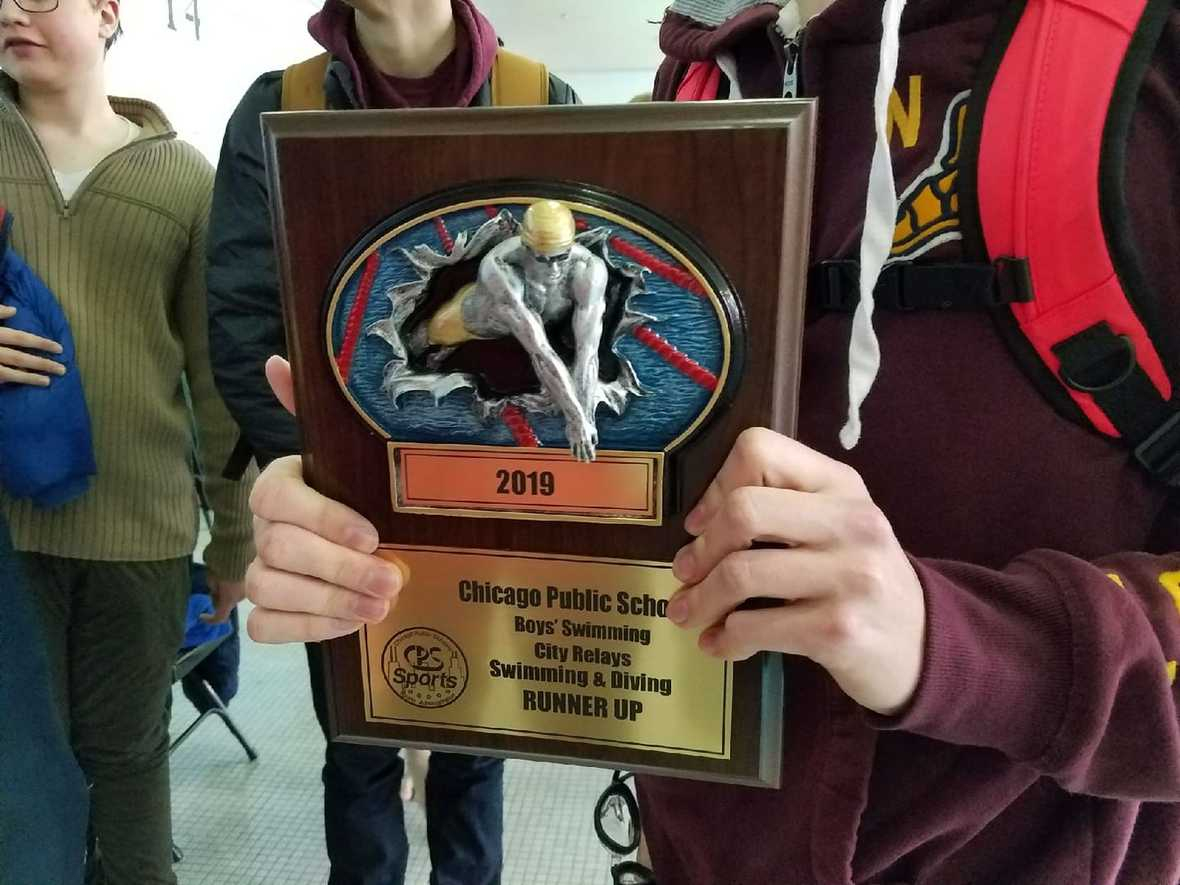# NCP Men's Swim Team Takes Second Place at the City Relay Championship Meet

In a field of 27 city high schools, the Northside Men's Swim Team placed second in the CPS City Relay Championship meet held Saturday, January 12, at Julian High School. Lane Tech won the championship.

Earlier in the week Northside defeated both Taft and Von Steuben in separate dual meets. Coming up soon is Senior Night, Tuesday, January 22, at 5:00 pm against Lincoln Park High School at the Northside pool.

Members of the team who participated in the meet included: Zheous Abalos, Osita Achufusi, Luis Acosta, Rocco Cappelletti, Daniel Claud, Justin Dihn, Kyle Hathaway, Octavio Hernandez, Lukas Hoffman, Ethan Massey, Ian Shimandle, Taber Skiba, Marcus Tschoe, Jovany Vasquez, and Collin Wentworth.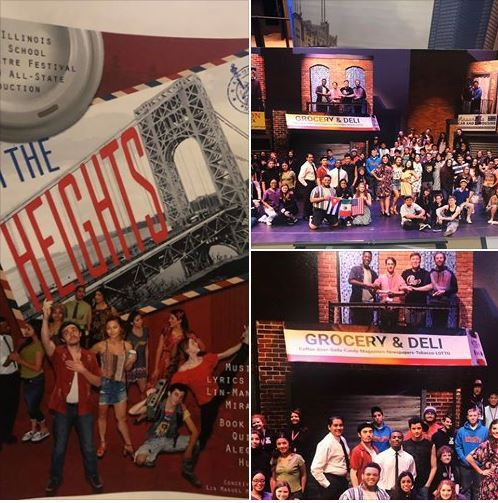# Northside Students Perform in the Illinois High School Theater Festival

Congratulations to the Northside Students who participated in the Illinois High School Theater Festival in Champaign. Their 5 performances of the musical In The Height were spectacular!

# Do you have news or team results to share with the Northside parent community?

 table div table+table+table+table+table+table+table+table+table+table+table+table+table+table+table+table+table+table+table+table+table+table+table+table+table+table+table+table+table+table+table+table+table+table+table+table+table+table+table+table+table+table+table+table+table+table+table+table+table+table+table+table+table+table+table+table+table+table+table+table div table{width:100%;padding:0}table div table+table+table+table+table+table+table+table+table+table+table+table+table+table+table+table+table+table+table+table+table+table+table+table+table+table+table+table+table+table+table+table+table+table+table+table+table+table+table+table+table+table+table+table+table+table+table+table+table+table+table+table+table+table+table+table+table+table+table+table div table img{width:96.23%;padding:0;float:none}table div table+table+table+table+table+table+table+table+table+table+table+table+table+table+table+table+table+table+table+table+table+table+table+table+table+table+table+table+table+table+table+table+table+table+table+table+table+table+table+table+table+table+table+table+table+table+table+table+table+table+table+table+table+table+table+table+table+table+table+table div table td{width:100%;padding:0 1.88% 18px}/* styles */# FON Connect

Friends of Northside uses a customer/constituent relationship management (CRM) system known as FON Connect that allows our parent volunteer organizations to work from one database. It is not a CPS database and is completely different and separate from the CPS Parent Portal and Northside's EPay website.

FON Connect serves as the NCP Family Directory and allows users to review their donation history, update their contact information, register for upcoming events, and more. Each parent has a separate account. Transactions, including donations, are recorded in the account of the parent who initiated the transaction or donation.

If you’re new to FON Connect, you'll need to set up a login and password. To do so:

 table div table+table+table+table+table+table+table+table+table+table+table+table+table+table+table+table+table+table+table+table+table+table+table+table+table+table+table+table+table+table+table+table+table+table+table+table+table+table+table+table+table+table+table+table+table+table+table+table+table+table+table+table+table+table+table+table+table+table+table+table+table+table div table{width:100%;padding:0}table div table+table+table+table+table+table+table+table+table+table+table+table+table+table+table+table+table+table+table+table+table+table+table+table+table+table+table+table+table+table+table+table+table+table+table+table+table+table+table+table+table+table+table+table+table+table+table+table+table+table+table+table+table+table+table+table+table+table+table+table+table+table div table img{width:96.23%;padding:0;float:none}table div table+table+table+table+table+table+table+table+table+table+table+table+table+table+table+table+table+table+table+table+table+table+table+table+table+table+table+table+table+table+table+table+table+table+table+table+table+table+table+table+table+table+table+table+table+table+table+table+table+table+table+table+table+table+table+table+table+table+table+table+table+table div table td{width:100%;padding:0 1.88% 18px}/* styles */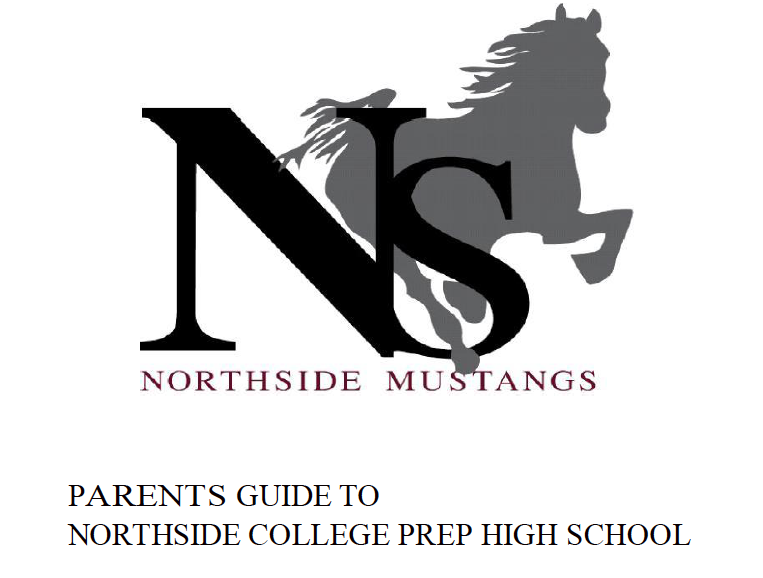# Navigating Northside

Navigating Northside, our parent handbook, is available on the school website under the Parents tab. Click HERE to access it directly.

 table div table+table+table+table+table+table+table+table+table+table+table+table+table+table+table+table+table+table+table+table+table+table+table+table+table+table+table+table+table+table+table+table+table+table+table+table+table+table+table+table+table+table+table+table+table+table+table+table+table+table+table+table+table+table+table+table+table+table+table+table+table+table+table+table div table{width:100%;padding:0}table div table+table+table+table+table+table+table+table+table+table+table+table+table+table+table+table+table+table+table+table+table+table+table+table+table+table+table+table+table+table+table+table+table+table+table+table+table+table+table+table+table+table+table+table+table+table+table+table+table+table+table+table+table+table+table+table+table+table+table+table+table+table+table+table div table img{width:96.23%;padding:0;float:none}table div table+table+table+table+table+table+table+table+table+table+table+table+table+table+table+table+table+table+table+table+table+table+table+table+table+table+table+table+table+table+table+table+table+table+table+table+table+table+table+table+table+table+table+table+table+table+table+table+table+table+table+table+table+table+table+table+table+table+table+table+table+table+table+table div table td{width:100%;padding:0 1.88% 18px}/* styles */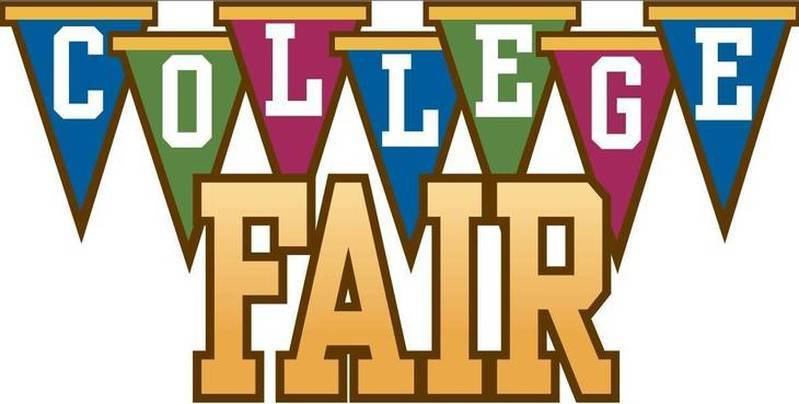# College Fair

Tuesday, March 5
6:00 - 8:00 pm
at NCP

Northside Prep, Disney II Magnet, and Von Steuben High School are hosting a joint College Fair.

Register today at www.strivefair.com.
If you are interested in volunteering for the fair, contact Monique Moore, NCP College Counselor, mcmoore6@cps.edu or (773)-534-3954 x26811.

# Upcoming College Visits at Northside

Thursday, January 17
Lincoln College (Lincoln), 10:38 am

Wednesday, January 23
IE University - Segovia, 12:26 pm

Friday, January 25
Truman State University, 9:48 am

# SAT Prep Course

The Academic Approach SAT Prep course for Northside College Prep students will begin Wednesday, January 16th, in preparation for the upcoming exam in April. The first day of class students will participate in a full-length practice SAT Test that will be approximately 3.5hours long. Please note, the Academic Approach spring 2019 SAT Prep Course dates are outlined below. If your family registered and submitted a payment for the course, an e-mail will be sent to you in the near future with additional details.

For more information, contact Monique Moore, Northside Prep College Counselor at mcmoore6@cps.edu or (773) 524-3954 x26811.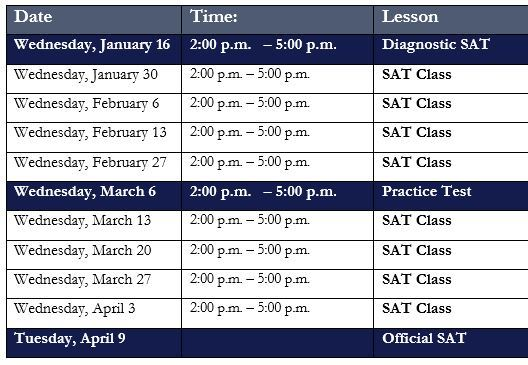# Naviance CCLR Live by Kaplan

## College, Career, and Life Readiness# NCP College Planning Handbook

This is a great resource that guides students through the entire college process. Click HERE to access a copy.

# NCP College Planning Additional Resources

Click on the links below to check out the additional resources on the Northside College Prep website:

 table div table+table+table+table+table+table+table+table+table+table+table+table+table+table+table+table+table+table+table+table+table+table+table+table+table+table+table+table+table+table+table+table+table+table+table+table+table+table+table+table+table+table+table+table+table+table+table+table+table+table+table+table+table+table+table+table+table+table+table+table+table+table+table+table+table+table+table+table+table+table+table+table+table div table{width:100%;padding:0}table div table+table+table+table+table+table+table+table+table+table+table+table+table+table+table+table+table+table+table+table+table+table+table+table+table+table+table+table+table+table+table+table+table+table+table+table+table+table+table+table+table+table+table+table+table+table+table+table+table+table+table+table+table+table+table+table+table+table+table+table+table+table+table+table+table+table+table+table+table+table+table+table+table div table img{width:96.23%;padding:0;float:none}table div table+table+table+table+table+table+table+table+table+table+table+table+table+table+table+table+table+table+table+table+table+table+table+table+table+table+table+table+table+table+table+table+table+table+table+table+table+table+table+table+table+table+table+table+table+table+table+table+table+table+table+table+table+table+table+table+table+table+table+table+table+table+table+table+table+table+table+table+table+table+table+table+table div table td{width:100%;padding:0 1.88% 18px}/* styles */# Help Northside When You Shop Online

## You can help our school with no extra cost to you.

AmazonSmile is a simple and automatic way to support Northside while you shop.

To choose Northside, please note that our AmazonSmile name is Friends of "North" "Side" (North Side - two words at AmazonSmile).

 table div table+table+table+table+table+table+table+table+table+table+table+table+table+table+table+table+table+table+table+table+table+table+table+table+table+table+table+table+table+table+table+table+table+table+table+table+table+table+table+table+table+table+table+table+table+table+table+table+table+table+table+table+table+table+table+table+table+table+table+table+table+table+table+table+table+table+table+table+table+table+table+table+table+table+table+table div table{width:100%;padding:0}table div table+table+table+table+table+table+table+table+table+table+table+table+table+table+table+table+table+table+table+table+table+table+table+table+table+table+table+table+table+table+table+table+table+table+table+table+table+table+table+table+table+table+table+table+table+table+table+table+table+table+table+table+table+table+table+table+table+table+table+table+table+table+table+table+table+table+table+table+table+table+table+table+table+table+table+table div table img{width:96.23%;padding:0;float:none}table div table+table+table+table+table+table+table+table+table+table+table+table+table+table+table+table+table+table+table+table+table+table+table+table+table+table+table+table+table+table+table+table+table+table+table+table+table+table+table+table+table+table+table+table+table+table+table+table+table+table+table+table+table+table+table+table+table+table+table+table+table+table+table+table+table+table+table+table+table+table+table+table+table+table+table+table div table td{width:100%;padding:0 1.88% 18px}/* styles */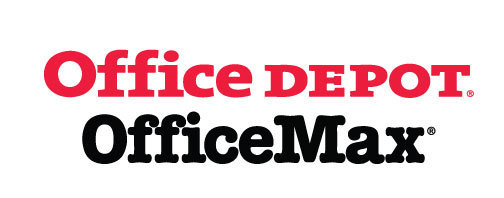# Give Back to Northside

Purchase school and office supplies at Office Depot and OfficeMax, and Northside can receive 5% back in credits or FREE supplies. Simply provide our school ID 70031171 at checkout, online or in stores. There’s no cost to you!

 table div table+table+table+table+table+table+table+table+table+table+table+table+table+table+table+table+table+table+table+table+table+table+table+table+table+table+table+table+table+table+table+table+table+table+table+table+table+table+table+table+table+table+table+table+table+table+table+table+table+table+table+table+table+table+table+table+table+table+table+table+table+table+table+table+table+table+table+table+table+table+table+table+table+table+table+table+table+table+table div table{width:100%;padding:0}table div table+table+table+table+table+table+table+table+table+table+table+table+table+table+table+table+table+table+table+table+table+table+table+table+table+table+table+table+table+table+table+table+table+table+table+table+table+table+table+table+table+table+table+table+table+table+table+table+table+table+table+table+table+table+table+table+table+table+table+table+table+table+table+table+table+table+table+table+table+table+table+table+table+table+table+table+table+table+table div table img{width:96.23%;padding:0;float:none}table div table+table+table+table+table+table+table+table+table+table+table+table+table+table+table+table+table+table+table+table+table+table+table+table+table+table+table+table+table+table+table+table+table+table+table+table+table+table+table+table+table+table+table+table+table+table+table+table+table+table+table+table+table+table+table+table+table+table+table+table+table+table+table+table+table+table+table+table+table+table+table+table+table+table+table+table+table+table+table div table td{width:100%;padding:0 1.88% 18px}/* styles */# Follow Us on Social Media

▪ Northside Prep Parents' Facebook Page, a closed group for parents, by parents, to share their knowledge of Northside
▪ Friends of Northside Facebook Page, for news everyone can view
 ▪ Northside Prep Parents' Facebook Page, a closed group for parents, by parents, to share their knowledge of Northside
 ▪ Friends of Northside Facebook Page, for news everyone can view
 table div table+table+table+table+table+table+table+table+table+table+table+table+table+table+table+table+table+table+table+table+table+table+table+table+table+table+table+table+table+table+table+table+table+table+table+table+table+table+table+table+table+table+table+table+table+table+table+table+table+table+table+table+table+table+table+table+table+table+table+table+table+table+table+table+table+table+table+table+table+table+table+table+table+table+table+table+table+table+table+table+table div table{width:100%;padding:0}table div table+table+table+table+table+table+table+table+table+table+table+table+table+table+table+table+table+table+table+table+table+table+table+table+table+table+table+table+table+table+table+table+table+table+table+table+table+table+table+table+table+table+table+table+table+table+table+table+table+table+table+table+table+table+table+table+table+table+table+table+table+table+table+table+table+table+table+table+table+table+table+table+table+table+table+table+table+table+table+table+table div table img{width:96.23%;padding:0;float:none}table div table+table+table+table+table+table+table+table+table+table+table+table+table+table+table+table+table+table+table+table+table+table+table+table+table+table+table+table+table+table+table+table+table+table+table+table+table+table+table+table+table+table+table+table+table+table+table+table+table+table+table+table+table+table+table+table+table+table+table+table+table+table+table+table+table+table+table+table+table+table+table+table+table+table+table+table+table+table+table+table+table div table td{width:100%;padding:0 1.88% 18px}/* styles */Northside’s website can be found HERE.

Friends of Northside's website can be found HERE.

 table div table+table+table+table+table+table+table+table+table+table+table+table+table+table+table+table+table+table+table+table+table+table+table+table+table+table+table+table+table+table+table+table+table+table+table+table+table+table+table+table+table+table+table+table+table+table+table+table+table+table+table+table+table+table+table+table+table+table+table+table+table+table+table+table+table+table+table+table+table+table+table+table+table+table+table+table+table+table+table+table+table+table+table div table{width:100%;padding:0}table div table+table+table+table+table+table+table+table+table+table+table+table+table+table+table+table+table+table+table+table+table+table+table+table+table+table+table+table+table+table+table+table+table+table+table+table+table+table+table+table+table+table+table+table+table+table+table+table+table+table+table+table+table+table+table+table+table+table+table+table+table+table+table+table+table+table+table+table+table+table+table+table+table+table+table+table+table+table+table+table+table+table+table div table img{width:96.23%;padding:0;float:none}table div table+table+table+table+table+table+table+table+table+table+table+table+table+table+table+table+table+table+table+table+table+table+table+table+table+table+table+table+table+table+table+table+table+table+table+table+table+table+table+table+table+table+table+table+table+table+table+table+table+table+table+table+table+table+table+table+table+table+table+table+table+table+table+table+table+table+table+table+table+table+table+table+table+table+table+table+table+table+table+table+table+table+table div table td{width:100%;padding:0 1.88% 18px}/* styles */